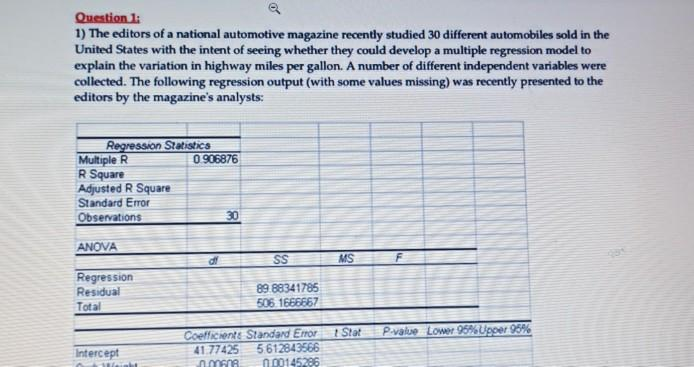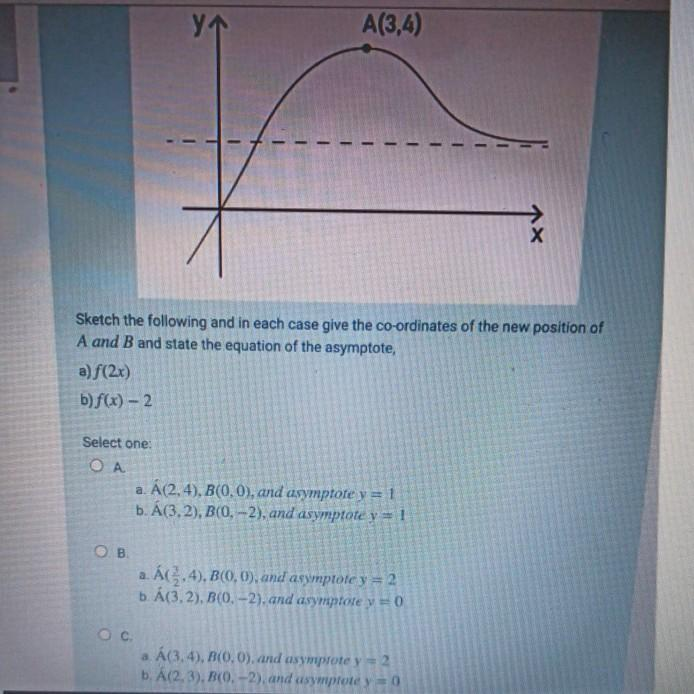# Question Question 1: 1) The editors of a national automotive magazine recently studied 30 different automobiles sold in the United States with the intent of seeing whether they could develop a multiple regression model to explain the variation in highway miles per gallon. A number of different independent variables were collected. The following regression output (with some values missing) was recently presented to the editors by the magazine's analysts: Regression Statistics Multiple R 0906876 R Square Adjusted R Square Standard Error Observations 30 ANOVA SS MS F Regression Residual Total 89 88341785 506 1666667 Star P-value Lower 95% Upper 95% Intercept Coefficiente Standard Emor 41.77425 5612843566 mana 0.00145296 У A(3,4) х Sketch the following and in each case give the co-ordinates of the new position of A and B and state the equation of the asymptote, a) f(2x) b) f(x) - 2 Select one: Ο Α. a. Á (2,4), B(0, 0), and asymptote y = 1 b. A(3,2), B(0, -2), and asymptote y = 1 OB a. ÁC.4), B(0, 0), and asymptote y = 2 b. A(3,2), B(O.-2). and asymptote y = 0 a Á(3.4), B(0,0), and asymprote y = 2 b. A(2,3), B(O.-2), and usymptote y